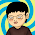## 2011年10月2日 星期日

### C# DateTime 與其他語言 DateTime 計算的注意事項

PHP、Java 等是以 Epoch 開始計算毫秒數，即 1970-01-01 00:00:00

``` long lServerTime = /* 取到 Server 告知的時間，例如 1317528009050 */ DateTime dtServer = TransDateTime(lServerTime); // 取得 Client、Server 相差的秒數 double diffSeconds = DateTime.Now.Subtract(dtServer).TotalSeconds; .... public static DateTime TransDateTime(long time) {     long cTime = (time * 10000) + (new DateTime(1970, 1, 1, 8, 0, 0).Ticks);     // 若要轉換的對像單位是秒而非毫秒，記得要多乘上 1000 如下     // long cTime = (time * 10000000) + (new DateTime(1970, 1, 1, 8, 0, 0).Ticks);     DateTime dTime = new DateTime(cTime);     return dTime; } ```

``` // 轉為正常毫秒計算的 Epoch long epochNow = Decimal.ToInt64(Decimal.Divide(DateTime.Now.Ticks - new DateTime(1970, 1, 1, 8, 0, 0).Ticks, 10000)) // 轉為秒數 long epochNow = Decimal.ToInt64(Decimal.Divide(DateTime.Now.Ticks - new DateTime(1970, 1, 1, 8, 0, 0).Ticks, 10000000)) // 此外若比較重視效率…也可以將上面的 DateTime.Now.Ticks - new DateTime(1970, 1, 1, 8, 0, 0).Ticks // 簡化為 DateTime.UtcNow.Ticks - 621355968000000000 ```

#### 3 則留言:

1.請問，不是從1970-1-1 0:0:0開始計算的嗎? 那為什麼會是減去new DateTime(1970,1,1,8,0,0), 而不是new DateTime(1970,1,1,0,0,0)呢?

1.是台北…我加了台北…

2.